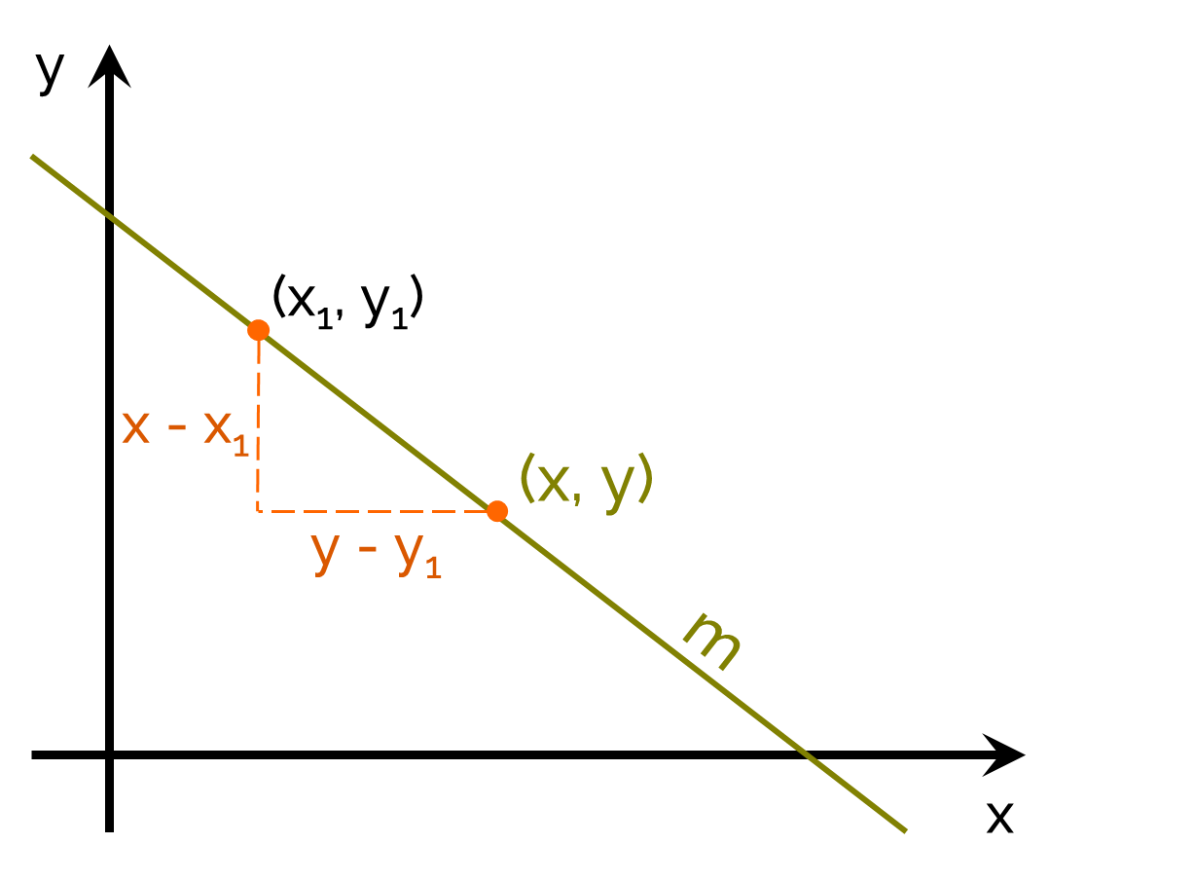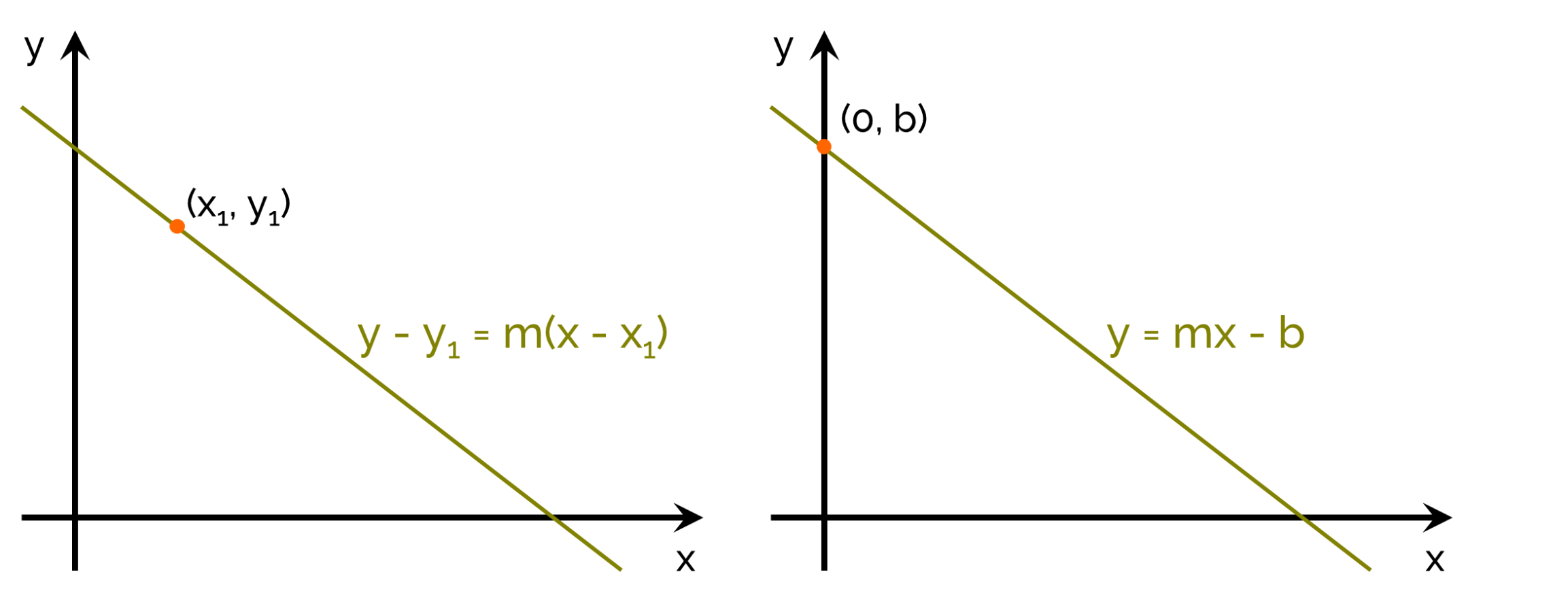# Point Slope Form Calculator

Created by Julia Żuławińska
Reviewed by Steven Wooding
Last updated: Apr 06, 2022

The point-slope form calculator will show you how to find the equation of a line from a point on that line and the line's slope. Soon, you will know what is point-slope form equation, and learn how is it different from the slope-intercept form equation. We also came up with two exercises, and we'll explain how to solve them in the last paragraph.

## What is a slope?

Let's start with the basics. What is the slope? The slope, also known as the gradient, is the marker of a line's steepness. If it's positive, it means the line rises. If it's negative - the line decreases. If it's equal to zero, the line is horizontal.

You can find the slope between two points by estimating rise over run - the difference in height over a distance between two points.So, slope formula is:

m = change in y / change in x = (y - y₁) / (x - x₁)

The point-slope form equation is a rearranged slope equation.

To find the gradient of non-linear functions, you can use the average rate of change calculator.

## What is the point-slope form?

There is more than one way to form an equation of a straight line. Point-slope form is a form of a linear equation, where there are three characteristic numbers - two coordinates of a point on the line, and the slope of the line. The point slope form equation is:

y - y1 = m * (x - x1),
where:

• x1, y1 are the coordinates of a point, and
• m is the slope.

Do you see the similarity to the slope formula? What you might not know is that it's not the only way to form a line equation. The more popular is the slope intercept form:

y = m * x + b,

where:

• m is the slope; and
• b is the intercept of the y-axis.

The truth is that this is nothing else than a more precise point-slope form. A straight line intercepts the y-axis in a point (0, b). If you choose this point - (0, b), as a point that you want to use in the point-slope form of the equation, you will get:

y - b = m * (x - 0), which is the same as y = m * x + b.

In the two graphs below, you can see the same function, only described with two different forms of a linear equation:## How to find the equation of a line with slope and coordinates of a point?

Let's have a look at two exercises, to understand the topic more clearly.

The slope of a line is 2. It passes through point A(2, -3). What is the general equation of the line?

1. Identify the point coordinates:
• x1 = 2,
• y1 = -3.
2. Identify the slope:
• m = 2
3. Input the values into the point slope form formula:
• y - y1 = m (x - x1)
• y - (-3) = 2(x - 2)
4. Simplify to get the general equation:
• y = 2x - 4 -3
• 0 = 2x - y - 7
And you have the answer. Now, you can check your result with our point-slope form calculator.

Let's solve an exercise with a more relatable subject.

Let's say you got a puppy. When you got him he was 14 pounds. It grew 0.2 pounds every day, and after 30 days, he was 20 pounds. Find the general equation of the puppy's growth.

1. The slope is the change of weight per day: m = 0.2
2. The characteristic point is 20 pounds on 30th day: (x1, y1) = (30, 20)
3. Now, input the values into the point-slope formula:

y - 20 = 0.2 * (x - 30)
4. Simplify the equation to get the general equation:
0 = 0.2x - y + 14

 💡 If you need to find a different point on your line click on the advanced mode button. Then, input one coordinate, and get the other.

And here you have it! We hope you enjoyed our point-slope form calculator! Before you go, check out more of our !

Julia ŻuławińskaPoint coordinates
x₁
y₁
Slope
m
People also viewed…

### Circumference

Use this free circumference calculator to find the area, circumference and diameter of a circle.

### Orthocenter

With this orthocenter calculator you'll quickly find out the coordinates of this important triangle center.

### Plastic Footprint

Find out how much plastic you use throughout a year with this plastic footprint calculator. Rethink your habits, reduce your plastic waste, and make your life a little greener.

### Sphere volume

Calculate the sphere volume, the volume of a spherical cap or a hemisphere, with this sphere volume calculator.